2021-10-03

## Recap

Prev: Principal Component Analysis

• Principal Components
• Interpretation

Now: Centroid-based clustering

• K-means
• Fuzzy c-means
• Geodemographic classification

"Clustering is an unsupervised machine learning task that automatically divides the data into clusters , or groups of similar items". (Lantz, 2019)

Methods:

• Centroid-based
• k-means
• fuzzy c-means
• Hierarchical
• Mixed
• bootstrap aggregating
• Density-based
• DBSCAN

## Example

Can we automatically identify the two groups visible in the scatterplot, without any previous knowledge of the groups?

# Prepared data
penguins_to_cluster <-
palmerpenguins::penguins %>%
dplyr::filter(
species %in%
) %>%
dplyr::filter(
!is.na(body_mass_g) |
!is.na(bill_depth_mm)
)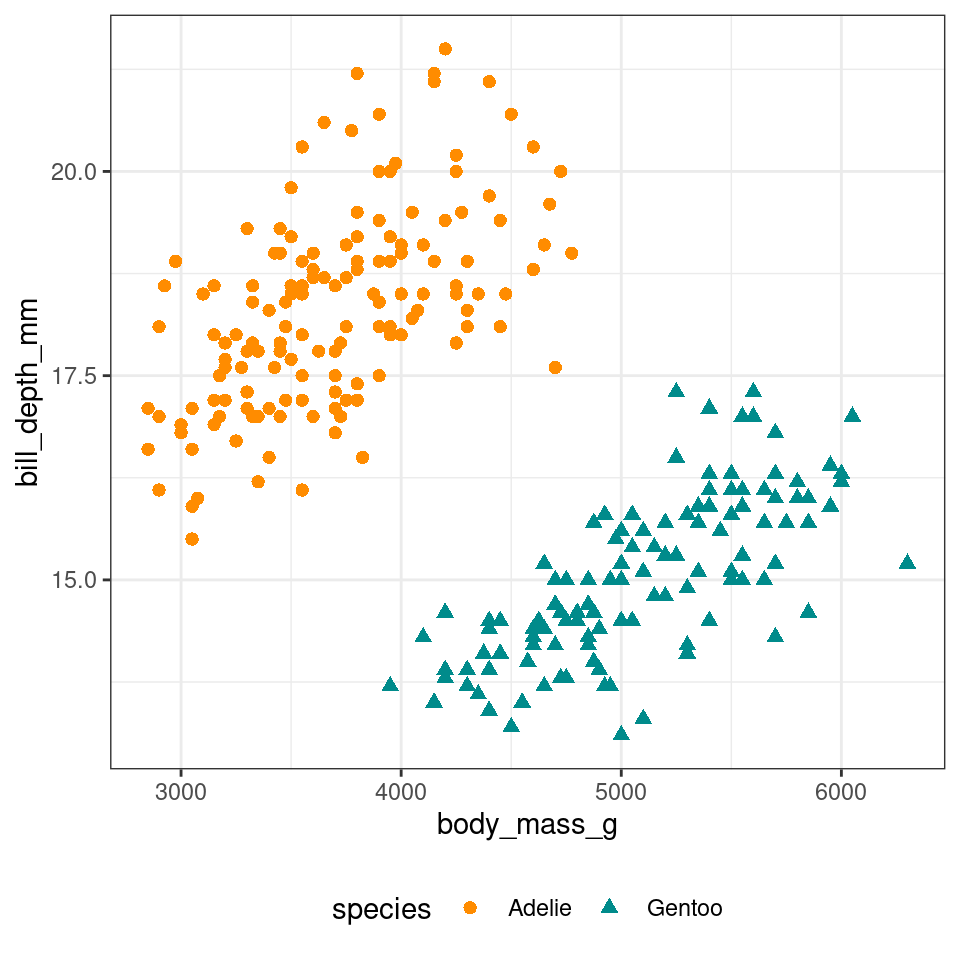## k-means algorithm

k-mean clusters $$n$$ observations ($$x$$) in $$k$$ clusters ($$c$$), minimising the within-cluster sum of squares (WCSS)

$WCSS = \sum_{c=1}^{k} \sum_{x \in c} (x - \overline{x}_c)^2$

Algorithm: k observations a randomly selected as initial centroids, then repeat

• assignment step: observations assigned to closest centroids
• update step: calculate means for each cluster, as new centroid

until centroids don’t change anymore, the algorithm has converged

## stats::kmeans

# Execute k-means
bm_bd_clusters <-
penguins_to_cluster %>%
dplyr::select(body_mass_g, bill_depth_mm) %>%
stats::kmeans(
centers = 2,  # number of clusters (k)
iter.max = 50 # max number of iterations
)

penguins_clustered_bm_bd <-
penguins_to_cluster %>%
)

## k-means result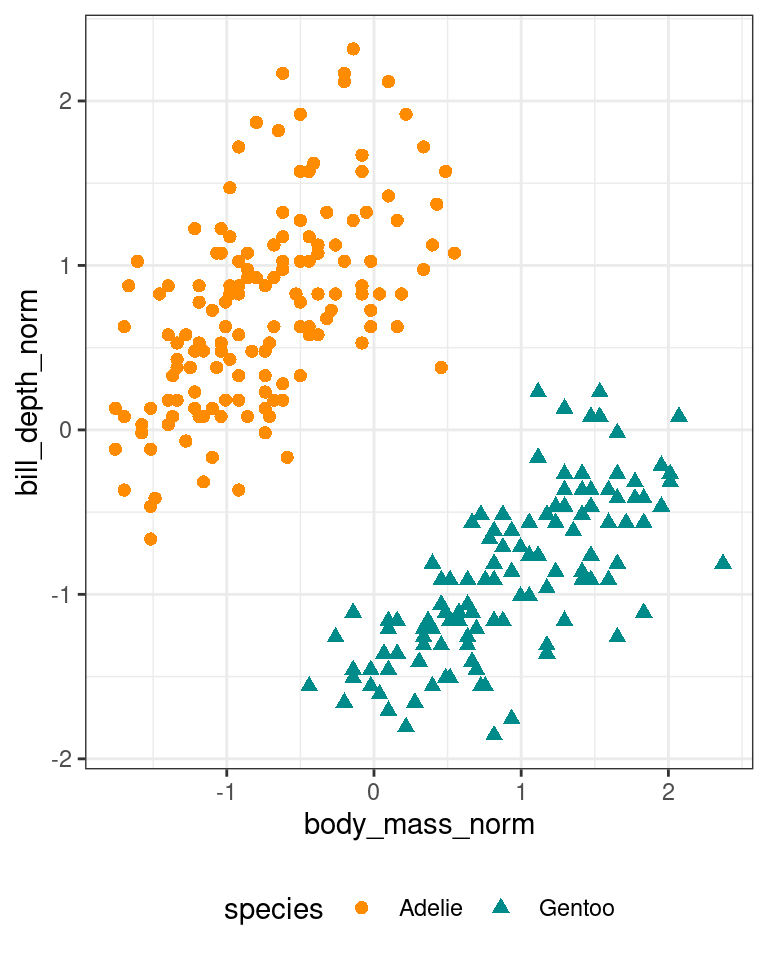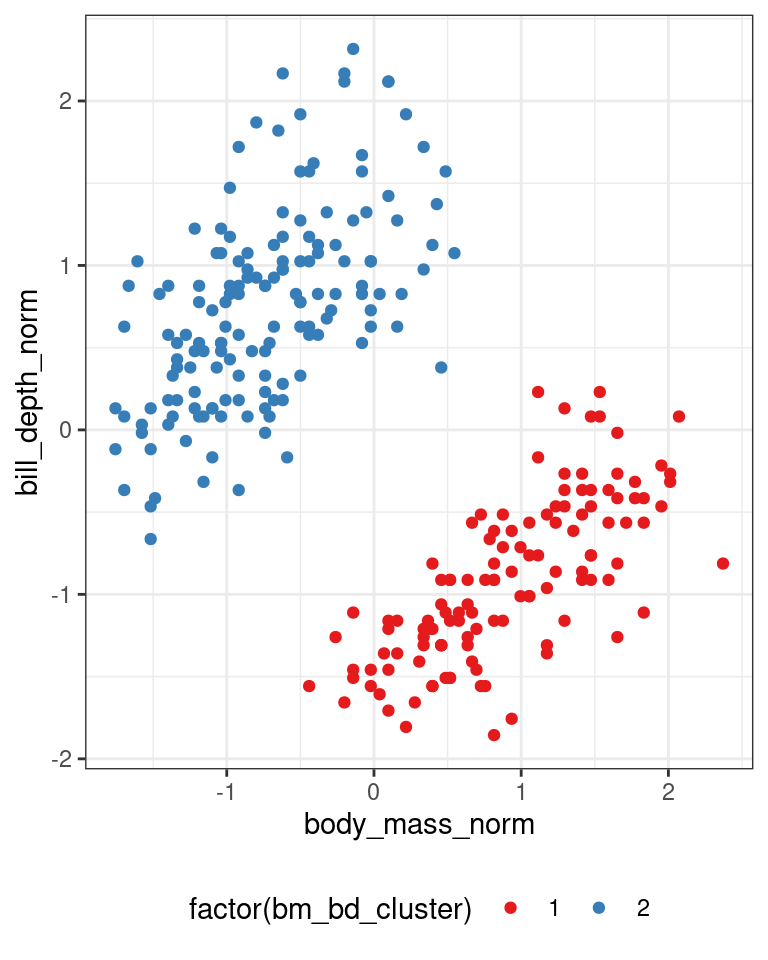## Limitations

K-means requires to select a fixed number of clusters in advance

Elbow method:

• calculate clusters for a range of number of clusters
• select the minimum number of clusters that minimises WCSS
• before increasing number of clusters leads minimal benefit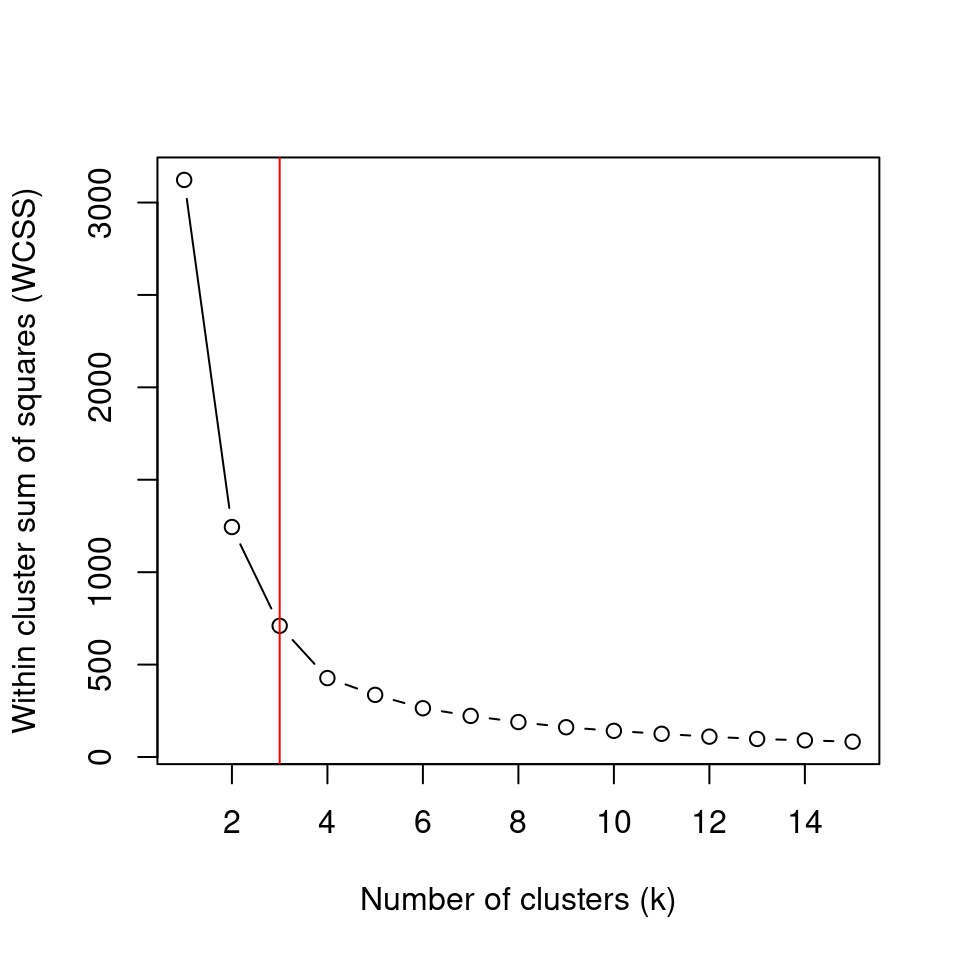Example for random data
generated to be in 3 clusters

## Fuzzy c-means

Similar to k-means but allows for "fuzzy" membership to clusters

Each observation is assigned with a value per each cluster

• usually from 0 to 1
• indicates how well the observation fits within the cluster
• i.e., based on the distance from the centroid
library(e1071)

bm_bd_norm_fclusters <- penguins_norm %>%
dplyr::select(body_mass_norm, bill_depth_norm) %>%
e1071::cmeans(centers = 2, iter.max = 50)

penguins_clustered_bm_bd_fuzzy <- penguins_norm %>%
tibble::add_column(bm_bd_fuzzy_cluster = bm_bd_norm_fclusters %$% cluster) ## Fuzzy c-means A “crisp” classification can be created by picking the highest membership value. • that also allows to set a membership threshold (e.g., 0.75) • leaving some observations without a cluster penguins_clustered_bm_bd_fuzzy <- penguins_clustered_bm_bd_fuzzy %>% tibble::add_column( bm_bd_fuzzy_cluster_membership = apply(bm_bd_norm_fclusters %$% membership, 1, max)
) %>%
dplyr::mutate(
bm_bd_crisp_cluster = ifelse(
bm_bd_fuzzy_cluster_membership < 0.75,
0, bm_bd_fuzzy_cluster
)
)

## Fuzzy c-means result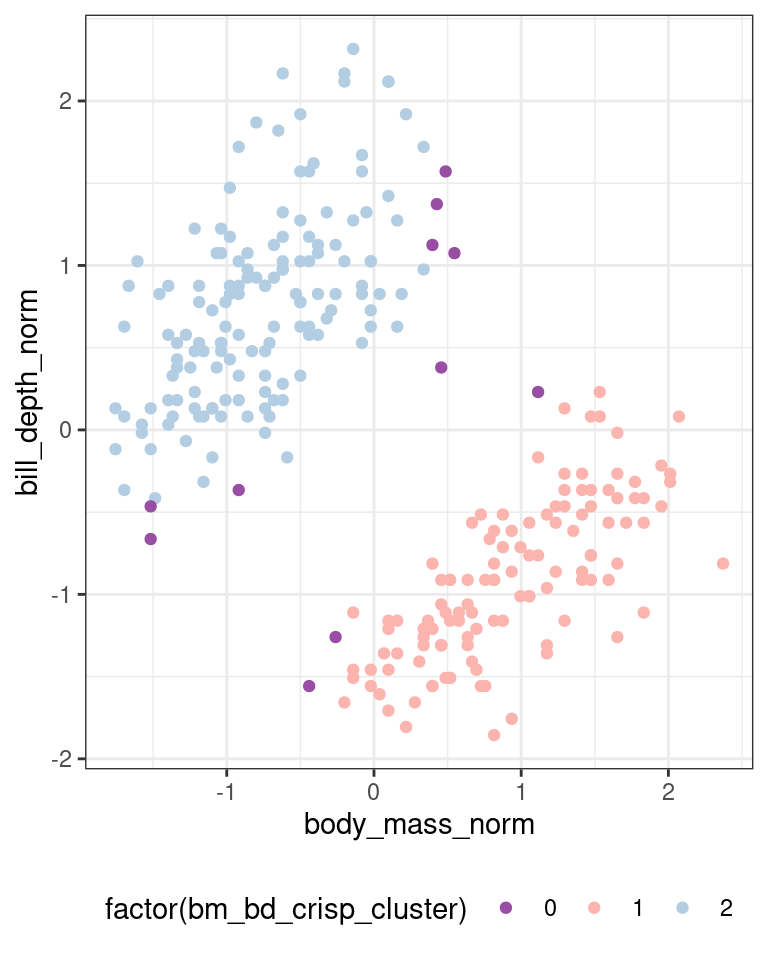## Geodemographic classifications

In GIScience, clustering is used to create geodemographic classifications such as the 2011 Output Area Classification from the UK Census 2011 (Gale et al., 2016)

• initial set of 167 prospective variables
• 86 were removed,
• 41 were retained as they are
• 40 were combined
• final set of 60 variables.
• k-means clustering approach to create
• 8 supergroups
• 26 groups
• 76 subgroups

## Summary

Centroid-based clustering

• K-means
• Fuzzy c-means
• Geodemographic classification

Next: Hierarchical and density-based clustering

• Hierarchical
• Mixed
• Density-based